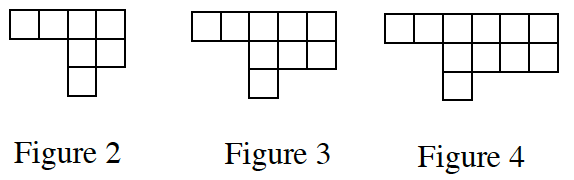### Home > CC4 > Chapter 1 > Lesson 1.2.2 > Problem1-49

1-49.

For the following tile pattern, find: 1-49 HW eTool (Desmos). Homework Help ✎

1. The growth rate of the pattern (the number of tiles added to each figure).

What is the growth rate? How many tiles is each figure increasing by? (This is the slope, or the $m$ value)

2. The number of tiles in Figure 8.

You could find the number of tiles in Fig. #5, then Fig. #6, until you reach Fig. #8. Or find the rule. Then plug '$8$' into the rule!

3. Sketch Figure 1.

• Work backwards with the pattern.Use the table in the eTool below to find the rule and graph the pattern shown.
Click the link at right for the full version of the eTool: Int1 1-49 HW eTool.